# Project 5.b 电阻网络

## 背景

• 串联 (Series): 将两个电阻首尾相连. 其等效电阻等于两个电阻的和.
• 并联 (Parallel): 将两个电阻并行排列. 其等效电阻等于倒数和的倒数.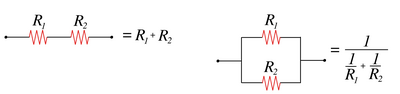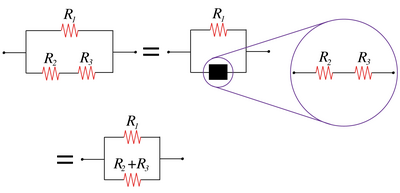## 如何表示网络

• $$R$$ 表示单个电阻器
• $$a$$ 为正数, 表示单个电阻器的取值
• $$N$$ 为电阻网络
• $$(-, N, N)$$ 表示两个电阻网络串联后的得到的网络
• $$(/, N, N)$$ 表示两个电阻网络并联后的得到的网络

1. $$N = (-, N, N)|(/, N, N)|R$$
2. $$R = a$$

1. 两个电阻为3和5的电阻器串联可以表示为: "(-, 3, 5)"
2. 两个电阻为3和5的电阻器并联可以表示为: "(/, 3, 5)"
3. 以下网络可以表示成为: "(-, (/, (/, 4, 8), 5), 3)"1. 以下网络可以表示成为: "(/, (-, (/, 6, 3), 2), (-, 3, 3))"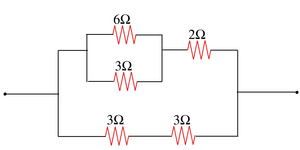## 实验内容

1. 写出下列网络的字符串表示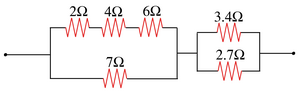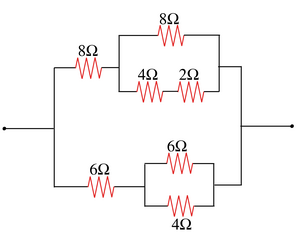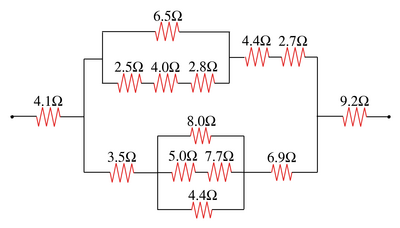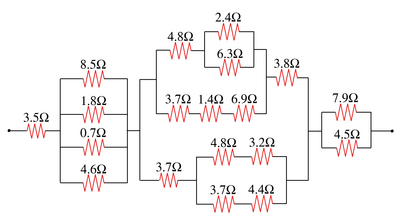1. 定义 Circuit 类用来表示电路网络.
public abstract class Circuit {
public abstract double resistance();
}

resistance() 返回该网络的等效电阻值. 给定网络的字符串表示, 构造相应的 Circuit 对象, 并输出它们的等效电阻值.

1. 修改 Circuit 类, 使得给定电源电压, 能够计算任意子网络两端的电势差以及流经该网络的电流.